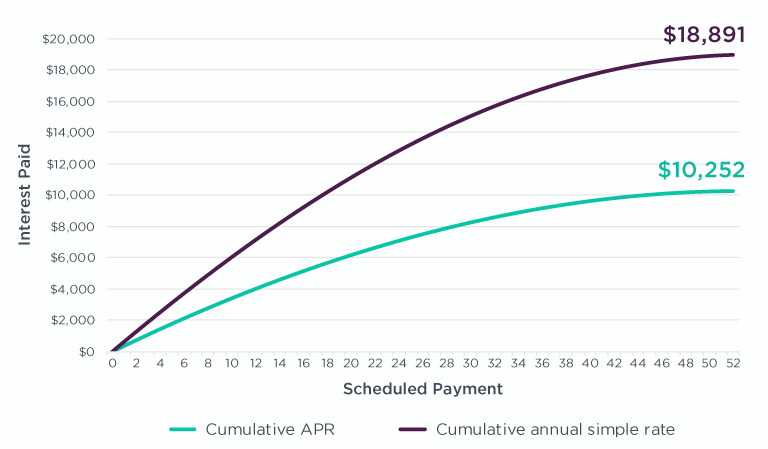# APR vs Simple Interest Rate – Why It’s Not So SimpleYour client’s business loan may be costing them more than you thought. Here's a short guide to help you understand the true cost difference between APR and simple interest.

######Illustration: The total cost of credit for a \$50,000 loan, over two years, showing the difference between 18.95% APR and 18.95% simple interest.

Business finance interest rates can be a minefield for both SMEs and brokers. While some lenders will be upfront and express interest rate in terms of APR (annual percentage rate), others might use annual simple interest, making the total cost of credit much more expensive.

### What is simple interest?

Simple interest is a basic way of looking at interest. Let’s consider an example. Using a simple interest rate of 18.95%, a \$50,000 loan over two years would cost \$18,950. The total principal and interest paid back is \$68,950. To calculate this, we can use the simple interest rate formula, which is Simple Interest = Principal x Rate x Time (in years).

So applying the simple interest rate formula to our example gives us:  \$50,000 x 18.95% x 2 = \$18,950.

Simple interest is calculated as if the entire loan amount is paid off in a lump sum at the end of the loan term. It doesn’t consider principal repayments. But most business loans have scheduled payments that reduce the amount outstanding over the loan term. So the presentation of a simple interest rate hides the real cost.

### What is APR?

The annual percentage rate (APR) is the rate used to calculate the true cost of the loan.

Consider our previous example. If the borrower gets the two-year \$50,000 loan and the interest rate is 18.95% APR, with a fortnightly repayment and fortnightly compounding, the total interest will be \$10,252. The principal and interest paid back will be \$60,252. Notice the difference between the total cost of credit with the simple interest rate and the APR. It’s \$8,698 (\$18,950 for the simple interest loan minus \$10,252 for the APR loan). So the true cost of the simple interest loan is much higher.

If you translate the loan quoted at 18.95% simple interest into APR and base it on fortnightly repayments, the APR for the loan is actually 33.55%.

### Comparing Simple Interest and APR Business Loans

The following table shows the differences between two business loans, one quoted in APR, the other using simple interest. Some lenders quote simple interest per annum, which slightly changes the number from the example in the previous sections.Considering the differences between simple interest and APR, you’ll want to make sure you’re asking the right questions upfront when dealing with lenders so you’re comparing apples to apples.

At Moula, transparency is one of our core values, which is why we worked with the Australian Finance Industry Association (AFIA) to develop the Online Small Business Lenders Code of Practice. This initiative included introducing SmartBox, a tool that helps you compare the most important metrics across different business loans from different lenders. These include APR, the total cost of credit, and a breakdown of fees.

Check out A Brokers Guide to Using Smart Box to Compare Business Loans to find out more.

#### Author: Team @ Moula

Business content for Australian SMEs. Sharing guides, growth hacks, and expert tips on finance, sales and marketing, and tech.

#### Get a repayment schedule

Close form panel

We’ll send the results to your inbox right now.

#### Email sent

Your email is on its way!

#### Something went wrong

Your repayment schedule email was not sent. Please call us on 1300 88 58 93 for assistance.